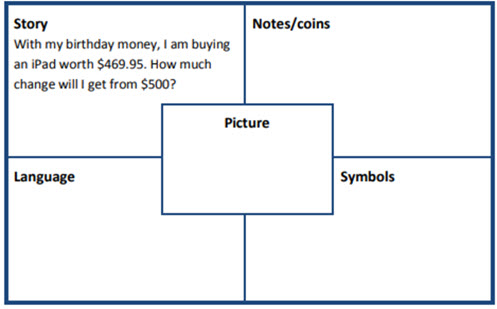# Tuckshop orders

Students make calculations with money.

Year level(s) Year 4
Audience Teacher
Purpose Teaching resource
Teaching strategies and pedagogical approaches Concrete Representational Abstract model, Culturally responsive pedagogies

## Curriculum alignment

Curriculum connections Numeracy
Strand and focus Number, Build understanding
Topics Addition and subtraction, Money and financial mathematics
AC: Mathematics (V9.0) content descriptions
AC9M4N08
Use mathematical modelling to solve practical problems involving additive and multiplicative situations including financial contexts; formulate the problems using number sentences and choose efficient calculation strategies, using digital tools where appropriate; interpret and communicate solutions in terms of the situation

Multiplicative strategies (P6)
Understanding money (P7)